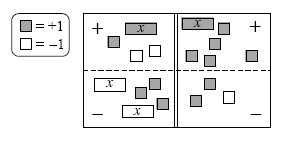### Home > AC > Chapter 4 > Lesson 4.1.2 > Problem4-13

4-13.Write the equation represented in the equation mat below.

1. Simplify as much as possible and then solve for $x$.

Right now the equation is:

$x+1+−2−(−2x+3)=x+5−(2+−1)$

Remove zero pairs.

Combine like terms.

Add $4$ to both sides.

Subtract $x$ from both sides.

Divide by $2$ on both sides.

$x+1+(−2)+2x+(−3)=x+5+−2+1$

$3x+(−4)=x+4$

$3x=x+8$

$2x=8$

$x=4$